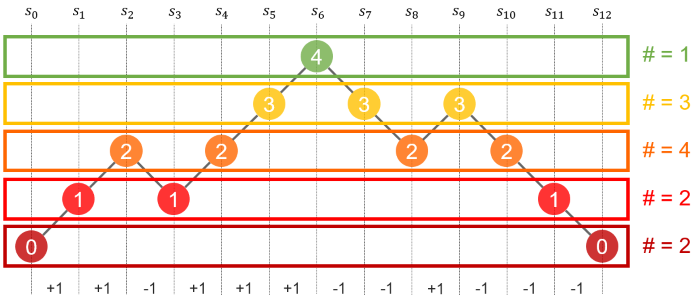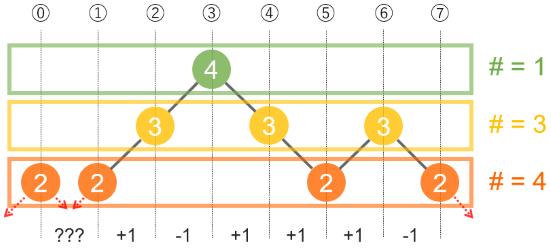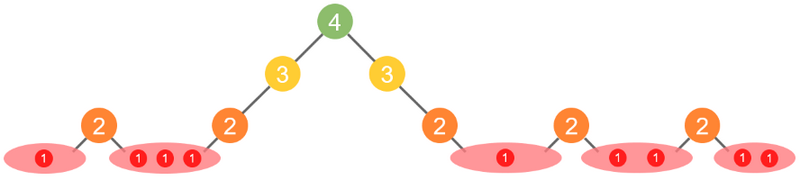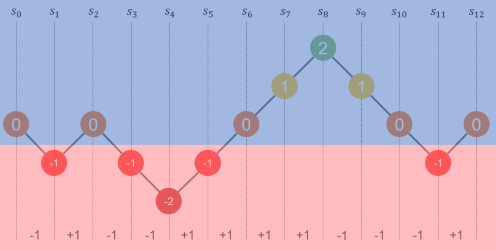Contest Duration: - (local time) (120 minutes) Back to Home
Official

## E - Zero-Sum Ranges 2 Editorial by evima

First, let us solve the following problem:

Given is a sequence of length $$n$$: $$a = (a_0, a_1, \dots, a_{n-1})$$.

How many pairs of integers $$l, r$$ $$(1 \leq l \leq r \leq N)$$ satisfy $$a_l + a_{l+1} + \cdots + a_{r-1} = 0$$?

We will briefly express the solution to this. Let $$s_i = a_0 + a_1 + \cdots + a_{i-1}$$. Then, $$a_l + a_{l+1} + \cdots + a_{r-1}$$ equals $$s_r - s_l$$, so the answer is the number of ways to choose two equal values from $$s_0, s_1, \dots, s_n$$.Now, let us go back to the original problem. Consider building the sequence of prefix sums “from top to bottom”:We will try a dynamic programming approach to count such sequences $$s_i$$. For now, let us only count such sequences that every $$s_i$$ is $$0$$ or greater. Let $$dp[size][cnt][hole]$$ be the number of sequences such that:

• the number of elements is $$size$$;
• the number of zero-sum ranges is $$cnt$$;
• the number of “holes” between two consecutive elements with the same value of $$s_i$$ is $$hole$$. (At the next level, we have to put at least one element in each hole, in addition to the left and right ends.)

Note that we do not have to store the levels of the sequences in this table because it is irrelevant to the transitions.

Then, considering the case we add $$x$$ elements at the next level, we have $$_{x-1}C_{hole+1}$$ ways to transit from each state $$(size, cnt, hole)$$ to $$(size + x, cnt + \frac{1}{2} x(x-1), x - (hole+2))$$. (Each of the $$x$$ elements should be added to one of the $$hole + 2$$ places: the holes and the two ends, and each place should have at least one element, so the number of ways to transit is equal to the number of sequences of $$(hole + 2)$$ positive integers totaling $$x$$).Since we have $$O(N^4)$$ states, we can fill the whole table in $$O(N^5)$$ time to count such sequences that every $$s_i$$ is $$0$$ or greater.

Using this table, we can find the number of all sequences: it is the sum of $$dp[x][y][z] \times dp[(2N+1)-x][K-y][z-1]$$ over all states $$(x, y, z)$$. (Alternatively, you can introduce to the table a new binary state representing $$level \geq 0$$ or $$level < 0$$.)Writer’s code: https://atcoder.jp/contests/arc117/submissions/21852196

Tester’s code: https://atcoder.jp/contests/arc117/submissions/21616984

posted:
last update: